# 1. Write molecular, total ionic, and net ionic equations for the following potassium sulfite silver aqueous...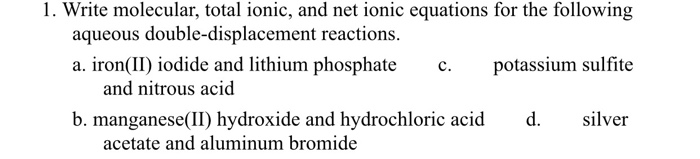1. Write molecular, total ionic, and net ionic equations for the following potassium sulfite silver aqueous double-displacement reactions. a. iron(II) iodide and lithium phosphate c. and nitrous acid b. manganese(II) hydroxide and hydrochloric acid d. acetate and aluminum bromide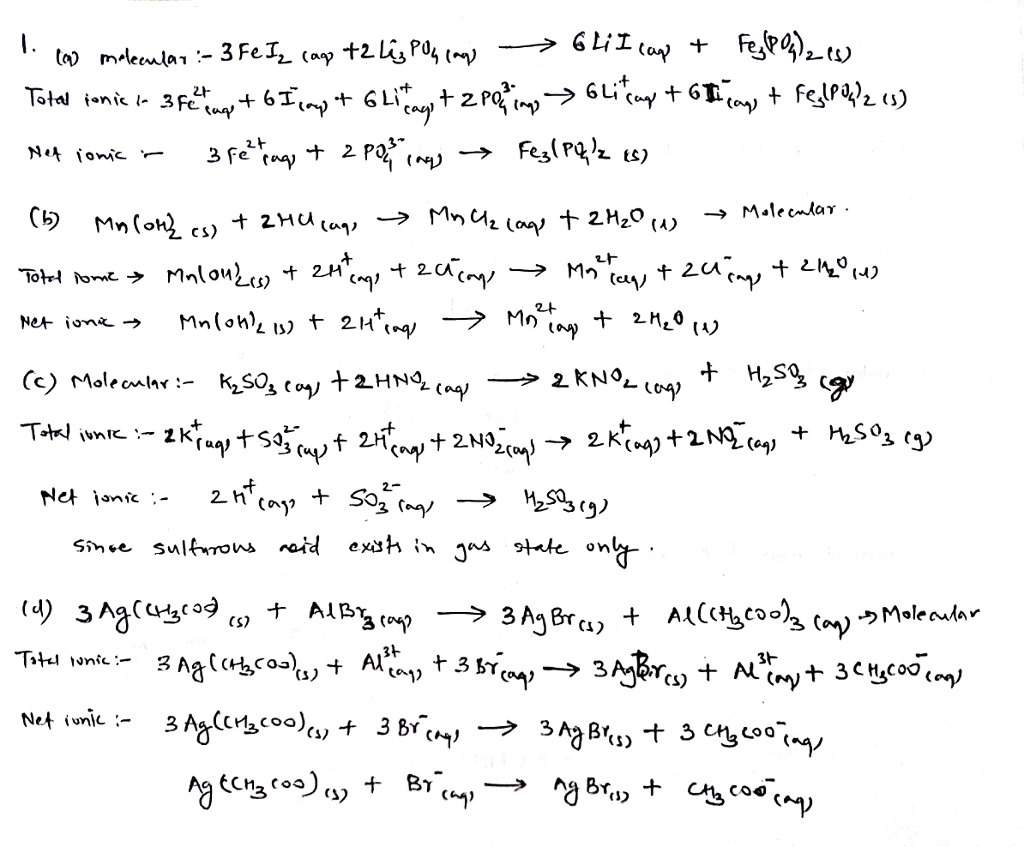##### Add Answer to: 1. Write molecular, total ionic, and net ionic equations for the following potassium sulfite silver aqueous...
Similar Homework Help Questions
• ### Grade (9 points) Write molecular, total lonic, and net ionic equations for the following aqueous double-...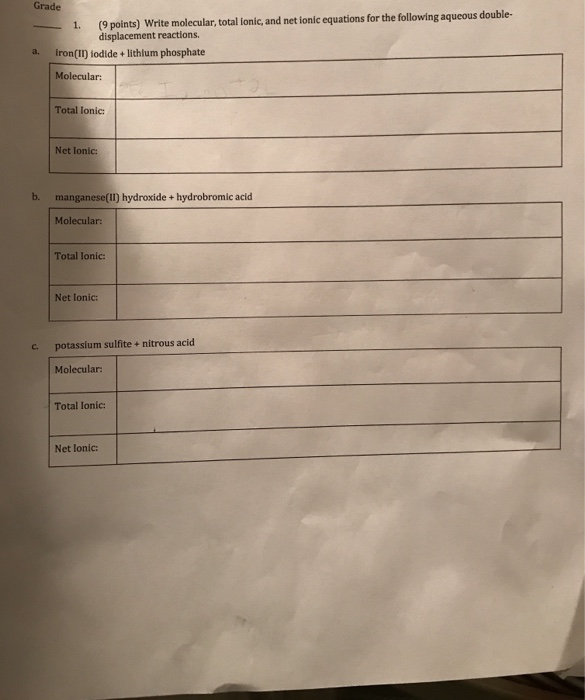Grade (9 points) Write molecular, total lonic, and net ionic equations for the following aqueous double- displacement reactions. a. Iron(II) iodide + lithium phosphate Molecular Total lonie: Net lonic: manganese(IT) hydroxide + hydrobromic acid Molecular: Total lonic Net Ionic: c. potassium sulfite + nitrous acid Molecular Total lonic: Net lonic: - 2. (12 points) Write balanced molecular, total ionic, and net ionic equations for the following aqueous single- displacement reactions. Also tell what is oxidized and what is reduced in...

• ### Identify each of the following aqueous reactions as double-displacement or single-displacement. Then write molecular, total ionic,...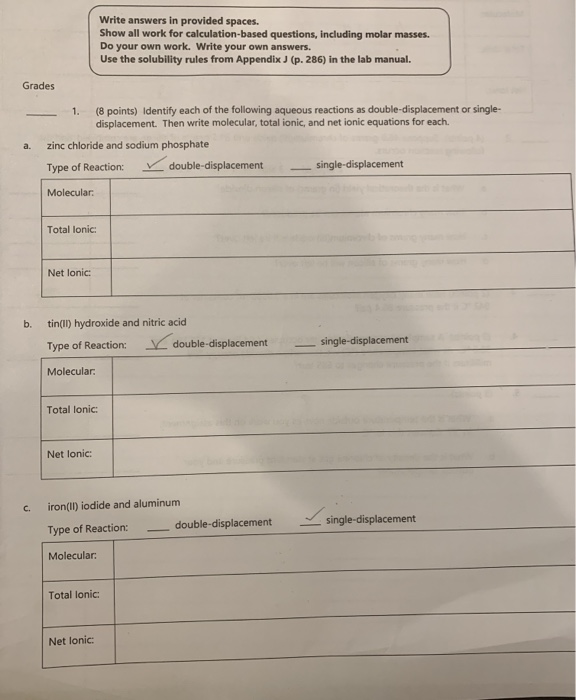Identify each of the following aqueous reactions as double-displacement or single-displacement. Then write molecular, total ionic, and net ionic equations for each. a. zinc chloride and sodium phosphate b. tin(II) hydroxide and nitric acid c. iron(II) iodide and aluminum d. chromium(III) perchlorate and boric acid Write answers in provided spaces. Show all work for calculation-based questions, including molar masses. Do your own work. Write your own answers. Use the solubility rules from Appendix J (p. 286) in the lab manual....

• ### Reaction 14: Aqueous iron(II) chloride + aqueous ammonium hydroxide Balanced Molecular Equation (from page 8): Complete...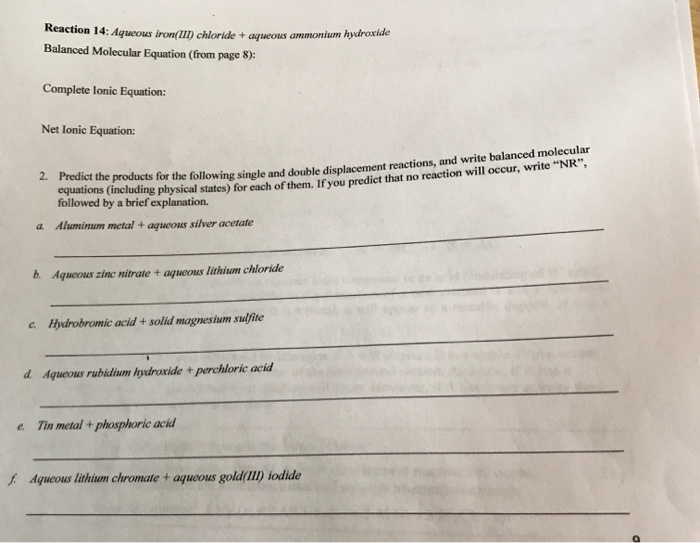Reaction 14: Aqueous iron(II) chloride + aqueous ammonium hydroxide Balanced Molecular Equation (from page 8): Complete lonic Equation: Net Ionic Equation: 2. Predict the products for the followine single and double displacement reactions, and wing single and double displacement reactions, and write balanced molecular squations including physical states) for each of them. If you predict that no reaction will occur, w na reaction will occur, write "NR", " followed by a brief explanation. a. Aluminum metal + aqueous silver acetate...

• ### write molecular, total ionic, and net ionic equations for hydrochloric acid and ammonium ca (5 points) Write molecu...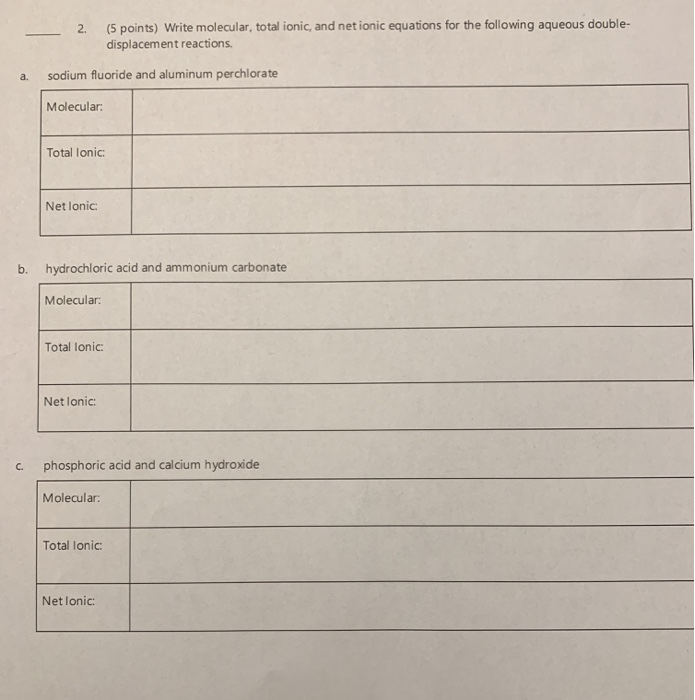write molecular, total ionic, and net ionic equations for hydrochloric acid and ammonium ca (5 points) Write molecular, total ionic, and net ionic equations for the following aqueous double displacement reactions. 2. sodium fluoride and aluminum perchlorate а. Molecular: Total lonic: Net lonic hydrochloric acid and ammonium carbonate b. Molecular: Total lonic: Net lonic phosphoric acid and calcium hydroxide C. Molecular: Total lonic Net lonic:

• ### Writing Balanced Molecular, Ionic, And Net Ionic Equations Write Balanced Net-Ionic Equations for the following Reactions...

Writing Balanced Molecular, Ionic, And Net Ionic Equations Write Balanced Net-Ionic Equations for the following Reactions in Aqueous Solution: 1.      Copper metal (Cu(s)) is immersed in an aqueous solution of silver nitrate (AgNO3). The solution turns light blue and a silver coating appears on the copper. 2.      Dilute solutions of antimony(III) chloride and sodium sulfide are mixed to give a precipitate. 3.      Dilute solutions of silver nitrate and potassium iodide are combined and give a yellow precipitate. 4.      Dilute solutions...

• ### Post Laboratory Questions 1. Write the equation for the decomposition of sulfurous acid. Usingl 2. Using...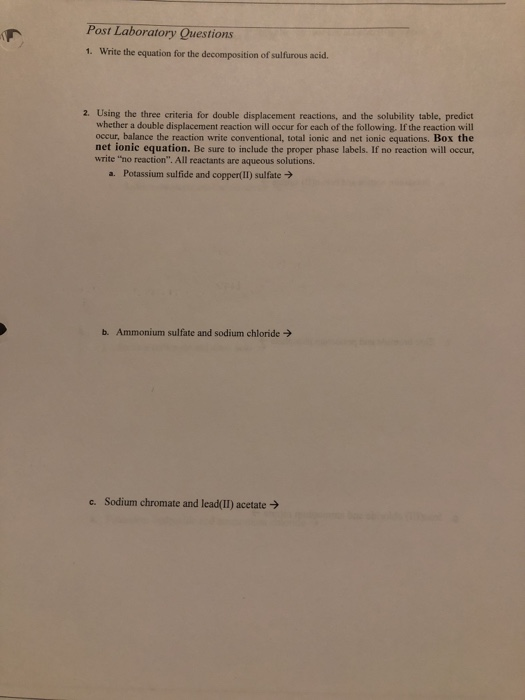Post Laboratory Questions 1. Write the equation for the decomposition of sulfurous acid. Usingl 2. Using the three criteria for double displacement reactions, and the solubility table. predict whether a double displacement reaction will occur for each of the following. If the reaction will occur, balance the reaction write conventional, total ionic and net ionic equations. Box the net ionic equation. Be sure to include the proper phase labels. If no reaction will occur. write "no reaction". All reactants are...

• ### Write balanced molecular, total ionic, and net ionic equations for the following aqueous single-displacement reactions....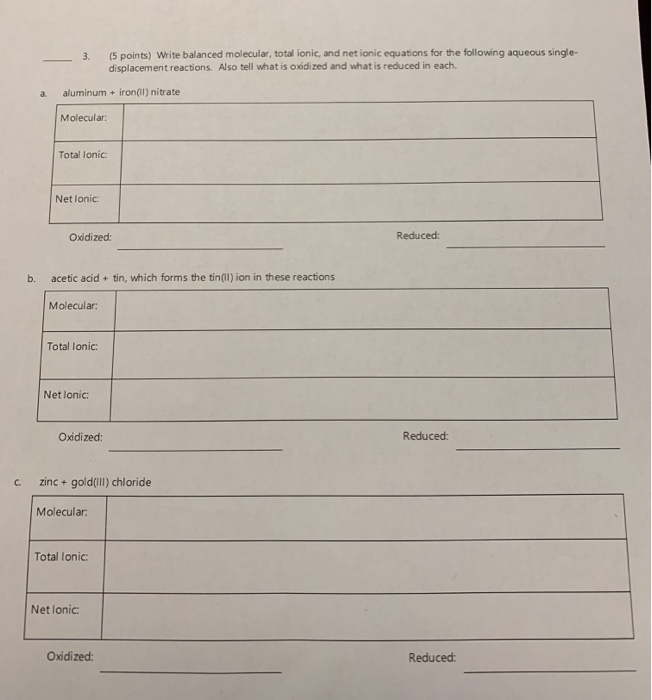Write balanced molecular, total ionic, and net ionic equations for the following aqueous single-displacement reactions. (5 points) Write balanced molecular, total ionic, and net ionic equations for the following aqueous single- 3. displacement reactions. Also tell what is oxidized and what is reduced in each. aluminum + iron(l) nitrate a. Molecular: Total lonic Net lonic Reduced: Oxidized: acetic acid+ tin, which forms the tin(ll) ion in these reactions b. Molecular: Total lonic: Net lonic: Reduced: Oxidized: zinc+ gold(iIlI) chloride C....

• ### whats the answers 1. Write molecular, complete ionic, and net jonic equations for the reactions that...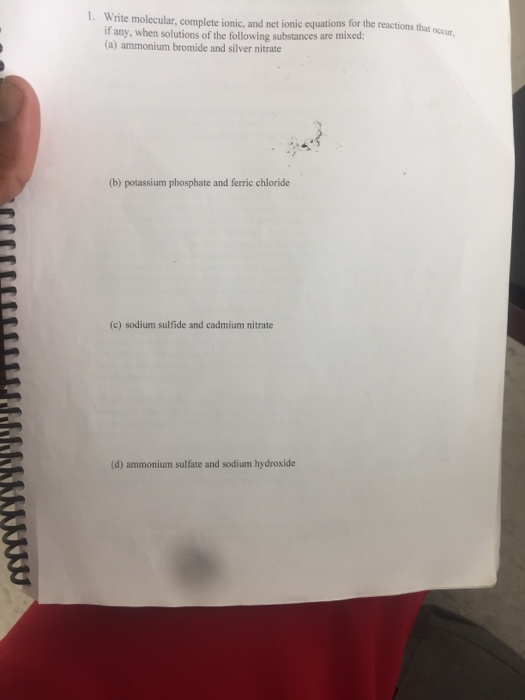whats the answers 1. Write molecular, complete ionic, and net jonic equations for the reactions that ove if any, when solutions of the following substances are mixed: (a) ammonium bromide and silver nitrate (b) potassium phosphate and ferric chloride (c) sodium sulfide and cadmium nitrate (d) ammonium sulfate and sodium hydroxide QUESTIONS: 1. Write molecular, complete ionic, and net ionic equations for the reactions that occur, if any, when solutions of the following substances are mixed: (a) ammonium bromide and...

• ### Write BALANCED NET IONIC EQUATIONS for the following additions of solutions 1. calcium bromide solution added to a...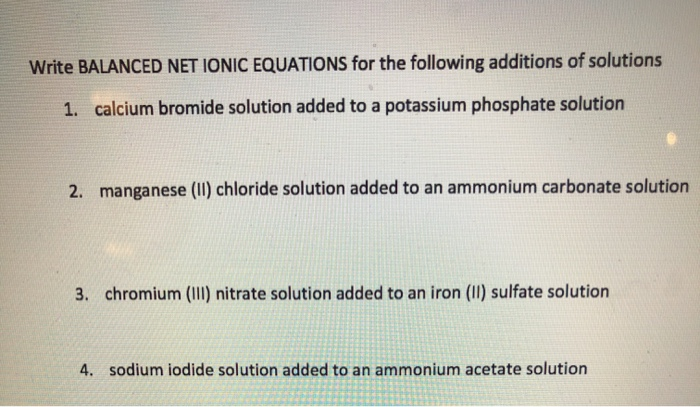Write BALANCED NET IONIC EQUATIONS for the following additions of solutions 1. calcium bromide solution added to a potassium phosphate solution 2. manganese (1) chloride solution added to an ammonium carbonate solution 3. chromium (III) nitrate solution added to an iron (II) sulfate solution 4. sodium iodide solution added to an ammonium acetate solution

• ### I was wondering If I was doing this correctly, (Other than the second equation where I mistaken Manganese for Magnesium)...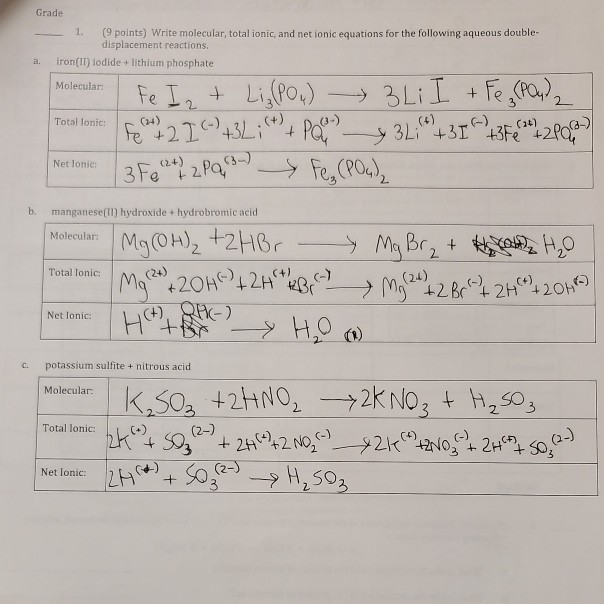I was wondering If I was doing this correctly, (Other than the second equation where I mistaken Manganese for Magnesium) Grade 1. (9 points) Write molecular, total ionic, and net ionic equations for the following aqueous double- displacement reactions fron(II) lodide +lithium phosphate a. Fe I LiPo) 3LiI +Fe (Pa,) Molecular: 2- ( () Pa Fe, (Pa) (4) Total lonic: Fe 3L+313Fe2P 2 Pa 3 Fe Net lonic: b. manganese(II) hydroxide + hydrobromic acid Mg(0H)2 t2H6r Ma 20H2 Molecular: 2...

Free Homework App